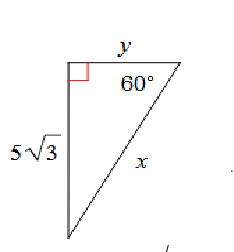GeoB - 30-60-90 wkst
Word problem. If the little leg is 6, what is the hypo? *
Word problem: If hypo is 20, what is little leg? *
Word problem: If hypo is 72, what is Big Leg? *
Word problem: If Little Leg is 7, what is Big Leg? *
Word problem: If big leg is 9, what is little leg? *
word problem: If Hypo is 18, what is little leg? *
Word problem: If big leg is 5 rad 3, What is hypo? *
find both *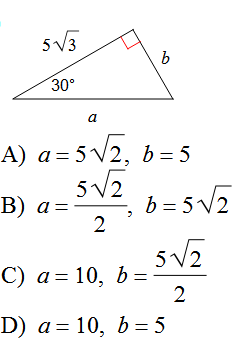Last name only *
period *
find x only *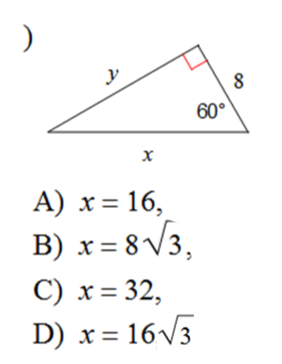find n *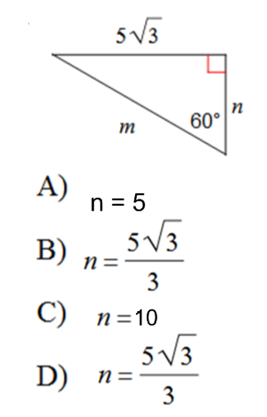find x *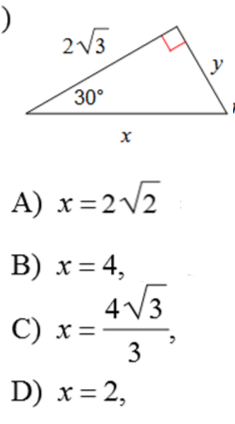Find b *find both *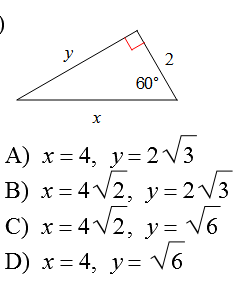find x *